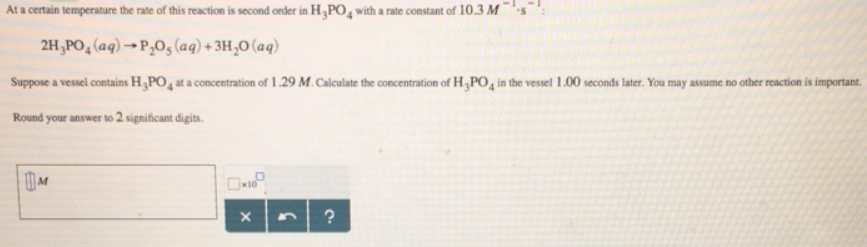# Problem: At a certain temperature the rate of this reaction is second order in H3PO4 with a rate constant of 10.3 M-1 • s-1. 2H3PO4 (aq) →  P2O5 (aq) + 3H2O (aq) Suppose a vessel contains H3PO4 at a concentration of 1.29 M. Calculate the concentration of H3PO4 in the vessel 1.00 seconds later. You may assume no other reaction is important. Round your answer to 2 significant digits.

###### FREE Expert Solution
93% (322 ratings)###### Problem Details

At a certain temperature the rate of this reaction is second order in H3PO4 with a rate constant of 10.3 M-1 • s-1

2H3PO4 (aq) →  P2O5 (aq) + 3H2O (aq)

Suppose a vessel contains H3PO4 at a concentration of 1.29 M. Calculate the concentration of H3PO4 in the vessel 1.00 seconds later. You may assume no other reaction is important.